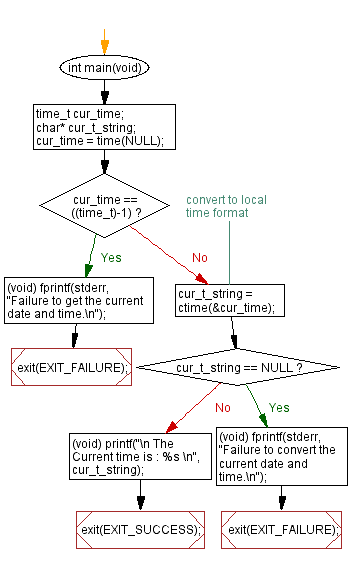﻿ C Program: Print the current date and time - w3resource

# C Exercises: Print the current date and time

## C Date Time: Exercise-1 with Solution

Write a program in C to print the current date and time.

Sample Solution:

C Code:

``````#include <time.h>
#include <stdio.h>
#include <stdlib.h>

int main(void)
{
time_t cur_time;
char* cur_t_string;
cur_time = time(NULL);
if (cur_time == ((time_t)-1))
{
(void) fprintf(stderr, "Failure to get the current date and time.\n");
exit(EXIT_FAILURE);
}
cur_t_string = ctime(&cur_time); //convert to local time format
if (cur_t_string == NULL)
{
(void) fprintf(stderr, "Failure to convert the current date and time.\n");
exit(EXIT_FAILURE);
}
(void) printf("\n The Current time is : %s \n", cur_t_string);
exit(EXIT_SUCCESS);
}
```
```

Sample Output:

``` The Current date and time is : Thu Aug 03 13:38:58 2017
```

N.B.: The result may vary for your current system date and time.

Flowchart:C Programming Code Editor:

What is the difficulty level of this exercise?

Test your Programming skills with w3resource's quiz.

﻿